## Triangle RST has vertices located at R (2, 3), S (4, 4), and T (5, 0). Part A: Find the length of each side of the triangle. Sho

Question

Triangle RST has vertices located at R (2, 3), S (4, 4), and T (5, 0).

Part A: Find the length of each side of the triangle. Show your work. (4 points)

Part B: Find the slope of each side of the triangle. Show your work. (3 points)

Part C: Classify the triangle. Explain your reasoning. (3 points)

Source
StylesFormat

in progress 0
1 month 2021-08-04T01:52:08+00:00 1 Answers 6 views 0

1. Given: The coordinate of R,S,T is (2,3), (4,4) and (5,0) respectively.

To find: Where we need to find the length of each side of the triangle RST

The slope of each side of the triangle RST.

and classify the triangle.

Solution:

Part A:

length of RS=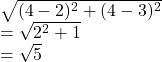Length of ST=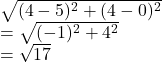Length of TR=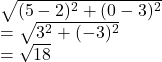Part B:

Slope of RS=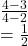Slope of ST=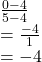Slope of TR=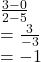Part C:

As all the side length of this triangle are unequal so this triangle is scalene.